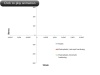# 从测量中获取结构力学的材料数据（第二部分）

2015年 5月 5日

### 非线性弹性材料COMSOL 共提供了 9 个非线性弹性材料模型，部分模型包含由几个参数决定的简单数学公式。其中一个材料模型特别适合用于处理应力-应变实验数据，即单轴数据。显而易见，该模型是基于测量数据进行分析。我们将分析该模型的设定：### 理论注解

• 平均应力（“压力”）或体积模量只是体积应变的函数。
• 剪应力或剪切模量只是剪应变的函数。

• 描述该依赖性的函数只能有一些非常具体的形式。
• 泊松比也必须为应变的函数，因此该函数将非常难以表达。\tau = G_S(\gamma) \cdot \gammaG_T(\gamma) = \frac{d \tau}{d \gamma} = G_S(\gamma) + \frac{d G_S(\gamma)}{d \gamma} \gamma

\tau = f(\gamma)

G_S(\gamma) = \frac{f(\gamma)}{\gamma}

\tau = C \gamma^n

G_s = C \gamma^n

COMSOL Multiphysics 的幂律模型中用到了更通用的第一个定义，在半对数绘图中，应变指数 n 与应力-应变曲线的斜率相关。

### 通过非线性弹性近似塑性• 显然，载荷只能持续上升。
• 如果同时承载了多个外部载荷，例如，压力载荷与热膨胀，它们通常与彼此不成比例，可能使局部应力变为非比例。
• 三维响应通常并不相同，即使单轴应力-应变曲线与非线性弹性模型和全弹塑性模型完全相同。对金属塑性而言，比如 von Mises 流动法则，塑性变形将保持体积不变。在对应的非线性弹性模型中，情况并非如此。

### 结束语

#### 评论 (2)

##### 留言##### Min Zhang
2022-06-08##### Qihang Lin
2022-06-22 COMSOL 员工